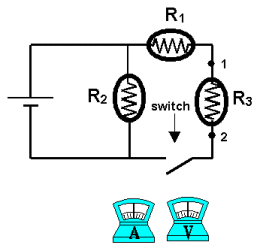Teaching Physics with the Physics Suite Edward F. Redish Problems Sorted by Type | Problems Sorted by Subject | Problems Sorted by Chapter in UP

Ammeters and Voltmeters

 In the figure at the right is shown a schematic diagram of a circuit consisting of a battery, 3 identical bulbs, and a switch. Assume that the battery is perfect (has no internal resistance) and the connecting wires are ideal (they have no resistance). In addition, you have an ideal ammeter, and an ideal voltmeter. The resistors are labeled R1, R2, and R3. In addition, two contact points are marked in the circuit and labeled 1 and 2. For each of the cases (a)-(f) below, assume you are starting from the configuration shown but with the switch closed and then perform the action described. Complete the sentence by selecting one of the following letters that describes how the indicated quantity changes: increases (I), remains the same (S), decreases but does not go to zero (D), becomes zero (0).1. If the switch is opened, the current through the resistor R2 _____.
2. If the switch is opened, the voltage drop across the resistor R1 _____.
3. If the switch is opened, the current flowing through the battery _____.
4. If the ammeter is connected between points 1 and 2 (in parallel with R3), the current in R3 _____.
5. If the voltmeter is connected between points 1and 2 (in parallel with R3), the current in R3 _____.
6. If the ammeter is connected into the circuit at point 1 (in series with R3), the current in R3 _____.

Note to the instructor: Students are often deeply confused about ammeters and voltmeters. Instead of seeing them as devices with physical properties, they may see them as "ideal and abstract" measuring devices, that is, whereever they are placed, they measure the current and voltage. Thinking through the cases of "incorrectly" attached ammeters and voltmeters sometimes helps.

Not finding what you wanted? Check the Site Map for more information.